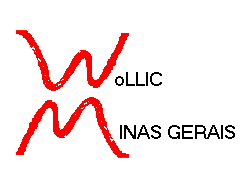WoLLIC'2003
10th Workshop on Logic, Language, Information and Computation

July 29th to August 1st, 2003

Ouro Preto, Minas Gerais, Brazil

Interest Group in Pure and Applied Logics (IGPL)
European Association for Logic, Language and Information (FoLLI)
Association for Symbolic Logic (ASL)
European Association for Theoretical Computer Science (EATCS)

Organisation
Centro de Informática, Universidade Federal de Pernambuco (CIn-UFPE)
Departamento de Matemática, Universidade Federal de Minas Gerais (DMAT/UFMG)
Departamento de Computação, Universidade Federal de Ouro Preto (DECOM/UFOP)

## Title and Abstract of Tutorial Lectures

Algorithmic Randomness and Derandomization
by Eric Allender (Department of Computer Science, Rutgers, the State University of NJ, USA)

Kolmogorov complexity is a tool to measure the information content of a string. Strings with high Kolmogorov complexity are said to be "K-random". The study of this notion of randomness has a long history and it has close connections with the theory of computability. The set of K-random strings has long been known to be undecidable.

Derandomization is a fairly recent topic in complexity theory, providing techniques whereby probabilistic algorithms can be simulated efficiently by deterministic algorithms.

In this tutorial, we will survey a few of the important developments in these two fields (with particular emphasis on derandomization and pseudorandom generators), and we will learn what these two fields have to do with each other. In particular, we will see what derandomization techniques tell us about what can be "efficiently" reduced to the set of K-random strings.

Generalized Quantifiers
by Lauri Hella (Department of Mathematics, Statistics and Philosophy, University of Tampere, Finland)

The idea of generalizing the usual existential and universal quantifiers is due to A. Mostowski (1957). He considered logical operators expressing cardinalities, like $Q_0 x$ and $Q_1 x$ which say that "there are infinitely many x" and "there are uncountably many x", respectively. Later Per Lindstrom (1966) generalized the notion of quantifier still further: according to his definition, any property of models of some fixed vocabulary can be taken as the interpretation of a quantifier. For example, if P is the property of graphs of being 3-colorable, and $Q_P$ is the corresponding quantifier, then the formula $Q_P xy \phi(x,y)$ says that the binary relation defined by $\phi(x,y)$ is a 3-colorable graph.

Extending a logic by generalized quantifiers is a minimal way of making undefinable properties definable. Indeed, if P is a property of models which is not definable in first order logic FO, then $FO(Q_P)$ is the least extension of FO, in which P is definable and which is closed under Boolean operations, first order quantifications and substituting relations by formulas. This fact has made generalized quantifiers a useful tool in studying th expressive power of various extensions of first order logic.

In this tutorial I will give a survey on the definability theory of generalized quantifiers. In particular, I will consider applications of quantifiers in the context of Finite Model Theory. I will also talk about some recent work on generalized second order quantifiers.

Implicit computational complexity
by Jean-Baptiste Joinet (Équipe de Logique Mathématique, Université Paris 7, France)

The proofs-as-programs paradigm, where computations are modelled by proofs conversion, offers a convenient theoretical framework for analysing properties of the computational dynamics of proofs/programs. In that frame, implicit computational complexity theory investigates computational complexity as a direct effect of the logical means involved (not as a by-product of ad hoc explicit external constraints). I will ouline the main recent approaches proposed in that field and will present how Linear Logic, having pull back to the logical level, the decomposition of dynamics into more fine grained operations, permits to design logically founded programming languages corresponding to several natural specific complexity classes.

Proof search foundations for logic programming
by Dale Miller (INRIA/Futurs/Saclay, and Laboratoire d'Informatique, École Polytechnique, France)

Sequent calculus is generally accepted as a foundation for logic programming, especially when the logic used is richer than first-order classical Horn clauses. I will outline a formal foundation of logic programming based on goal-directed sequent calculus provability, and will illustrate how higher-order quantification and linear logic all fit well into this foundation. Numerous examples of logic programming specifications using higher-order linear logic will be presented.

Iterated theory change
by Hans Rott (Institut für Philosophie, Universität Regensburg, Germany)

It is well known that early models of theory revision developed by Alchourrón, Makinson and Gärdenfors in the 1980s could account only for single changes of belief. Things changed only later when various authors addressed the problem of iterated theory change. The tutorial offers an overview over various ideas and concepts for iterated theory change that were developed in the past ten years or so (with a certain emphasis on the speaker's own work). Topics include
(1) revisions of "belief bases"
(2) revisions of "belief states"
(3) revisions by sequences
(4) revisions by comparisons